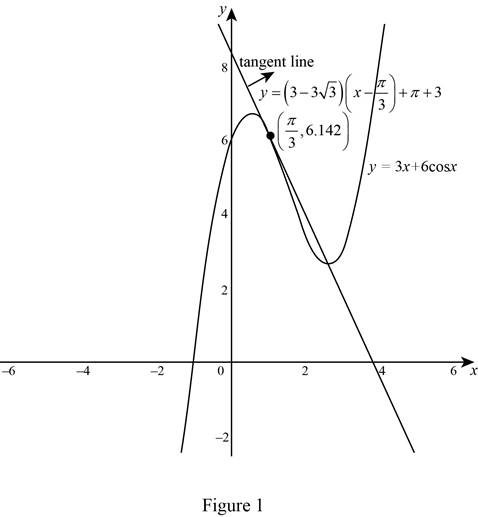# The equation of the tangent line to the curve at the point.### Single Variable Calculus: Concepts...

4th Edition
James Stewart
Publisher: Cengage Learning
ISBN: 9781337687805### Single Variable Calculus: Concepts...

4th Edition
James Stewart
Publisher: Cengage Learning
ISBN: 9781337687805

#### Solutions

Chapter 3.3, Problem 24E

(a)

To determine

## To find: The equation of the tangent line to the curve at the point.

Expert Solution

The equation of the tangent line to the curve y=3x+6cosx at (π3,π+3) is y=(333)x+3+π3_.

### Explanation of Solution

Given:

The equation of the curve is, y=3x+6cosx and the point is (π3,π+3).

Derivative rules:

(1) Constant Multiple Rule: ddx[cf(x)]=cddxf(x)

(2) Power Rule: ddx(xn)=nxn1

(3) Sum Rule: ddx[f(x)+g(x)]=ddx[f(x)]+ddx[g(x)]

Formula used:

The equation of the tangent line at (x1,y1) is, yy1=m(xx1) (1)

where, m is the slope of the tangent line at (x1,y1) and m=dydx|x=x1.

Calculation:

The derivative of y is dydx, which is obtained as follows,

dydx=ddx(y) =ddx(3x2x3)

Apply the sum rule (3)

ddx[3x+6cosx]=ddx[3x]+ddx[6cosx]

Apply the constant multiple rule(1).

ddx[3x+6cosx]=3ddx[x]+6ddx[cosx]

Apply the power rule (2)and simplify the expressions,

ddx[3x+6cosx]=3(1x11)+6(sinx)=36sinx

Therefore, the derivative of the function y=3x+6cosx is 36sinx_.

The slope of the tangent line at (π3,π+3) is,

m=dydx|x=π3 =36sinπ3           (Qsinπ3=32)=36(32)=333

Thus, the slope of the tangent line at (π3,π+3) is m=333_.

Substitute (π3,π+3) for (x1,y1) and 333 for m in equation (1),

y(π+3)=(333)(xπ3)yπ3=(333)x(333)π3yπ3=(333)xπ+3πy3=(333)x+3π

Add 3 on both sides and simplify further,

y3+3=(333)x+3π+3y=(333)x+3π+3

Therefore, the equation of the tangent line to the curve y=3x+6cosx is y=(333)x+3+3π.

(b)

To determine

Expert Solution

### Explanation of Solution

Given:

The curve is y=3x+6cosx and the tangent line at (π3,π+3) is y=(333)x+3+3π.

Graph:

Use the online graphing calculator to draw the graph of the functions as shown below in Figure 1.From Figure 1, it is observed that the equation of the tangent line touches the curve y=3x+6cosx at the point (π3,π+3).

### Have a homework question?

Subscribe to bartleby learn! Ask subject matter experts 30 homework questions each month. Plus, you’ll have access to millions of step-by-step textbook answers!